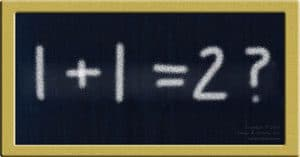1-330-777-0094
[email protected]
26 Aug 2010

This entry is part 2 of 9 in the series Challenging Assumptions

Challenging assumptions behind 1+1=2 makes for a great case study. For instance, we know that looking at the same thing from other viewpoints can change that thing. This happens when one looks at 1+1=2 in different ways. Answers change.

What make it a great case study are its simplicity and apparent unalterable truth. One can change the views very simply to get simply understood answers. In short, 1+1=2 really shows how fickle facts can be. It matters who is looking at them and from what point of view.Challenging assumptions behind 1+1=2 trains our brain to spot hidden assumptions and makes us better problem solvers.

## Three Examples Challenging Assumptions Behind 1+1=2

Therefore, let’s first look at the assumptions behind 1+1=2. Then, we’ll change them three times. That will give us three other answers. So, that gives us four true expressions of 1+1:

1. 1+1=2
2. 1+1=10
3. 1+1=11
4. 1+1=1

The reason why 1+1=2 is that that’s the rule of a numeric language called Base 10. Our daily lives work under the assumption that any number we see uses this language. We assume it so much that we no longer know we are assuming.

## Challenging The Assumption Of Numeric Language

The second expression, 1+1=10, is challenging assumptions behind 1+1=2 by changing the language of numbers. In this case, it’s not Base 10. It’s Binary. That’s the numeric language computers use. Only two numbers, 0 and 1, exist. In computers that means “off” and “on.”

In this language, 1+1=2 shows up as 1+1=10. All we did was change the language of numbers. It’s similar to how the word “run” changes depending on how we use it. We’re just changing context, a common way of changing how things work.

## Challenging The Assumption That Numbers Are Numbers

An easy way of challenging assumptions behind 1+1=2 is to say they aren’t numbers. They’re shapes. So the third expression says that we’re adding one shape to another. That give us two. By changing to a different shape we can see this more easily. Imagine adding one club to another. That gives us ♣ + ♣ = ♣♣. From this view then, 1+1=11 says the same thing.

Another way to challenge looking at 1+1 as numbers is to look at them symbolically. For instance, consider that one man plus one woman equals one couple. In short, we are challenging what each number represents. Thus, we can get 1+1=1. As other examples, consider that to get a single drink one might have to combine one part A and one part B. Here’s one more. One day equals one period of daylight and one of darkness.

Thus, by challenging assumptions behind1+1=2, this case study trains our minds in two ways. First, it trains it to spot assumptions. Then, it trains it to change them so we look at things from a different viewpoint. This not only helps us spot problems and solutions. It also helps to open many other doors that we would not otherwise see locked in by one view.

Series Navigation<< How Challenging Assumptions Every Day Improves Problem SolvingChallenging Assumptions Example >>

### 5 Responses

1.This completely reminds me of an LSAT style question

1.Never thought of that, James, but it does! ~Mike

2.Two others:

1+1=1 –> One person and one person on one team doing everything together

1+1=3+ –> The work of one person and the work of one person on a team equals the work of three or more people when working effectively as a team

1.Mike Lehr

Thank you very much for the additions, John. I appreciate it! I’ll have to include in a sequel to this post. Thank you again for stopping by for a visit and connecting on LinkedIn. ~Mike

1.